# Number field

(diff) ← Older revision | Latest revision (diff) | Newer revision → (diff)
A field consisting of complex (e.g., real) numbers. A set of complex numbers forms a number field if and only if it contains more than one element and with any two elementsandtheir differenceand quotient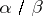(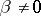). Every number field contains infinitely many elements. The field of rational numbers is contained in every number field.
Examples of number fields are the fields of rational numbers, real numbers, complex numbers, or Gaussian numbers (cf. Gauss number). The set of all numbers of the form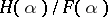,, forms a number field,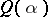, whereis a fixed complex number and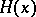and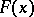range over the polynomials with rational coefficients.
An algebraic number fieldof degreeis an extension of degreeof the fieldof rational numbers. Alternatively, a number fieldis an algebraic number field (of degree) if every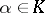is the root of a polynomial (of degree at most) over. A number field that is not algebraic is called transcendental. (Cf. also Algebraic number theory; Extension of a field; Transcendental extension.)